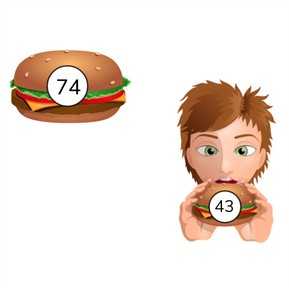Subtraction to 100

# Subtraction to 1008,000 schools use Gynzy92,000 teachers use Gynzy1,600,000 students use Gynzy

## General

Students learn to subtract numbers to 100.

2.NBT.B.5

## Relevance

It is important to be able to subtract numbers to 100, so you can determine how many you have left.

## Introduction

Ask students to use the number line shown on the interactive whiteboard to solve the given subtraction problems. You can ask them to explain their thinking as they solve the problem.

## Development

Discuss with students the importance of subtracting numbers to 100. The learning goal will be presented in three ways, visually, abstract, and in story form. You can select the method via the blue menu on the bottom right of the page and select the method that best fits your classroom needs.
The visual method starts by counting the number of objects on the interactive whiteboard that represent each number in the subtraction problem. Explain that you will take the second number away from the first. Show that you start by taking the tens away from 76. Take 60 away. You now have 16. Next take away 3 more. Your difference is 13. You can solve the next problem together with the class. Check that students are able to solve the math problem. In the abstract, show students that they can subtract using jumps on a number line. Show that you first take away the tens, and then the ones. Explain the two other ways of subtracting the same math problem without using the number line. Emphasize that students may select which method to use when subtracting, it is all about what works best for them. Solve a few more abstract subtraction problems individually or in pairs. Finally refresh the steps of solving a story problem with the students. First they must determine what kind of math problem it is, determine which numbers are important, say or write the math problem, and then solve the math problem. Do a story problem together and then have students solve a story problem individually or in pairs.

Check that students are able to subtract numbers to 100 by asking the following questions:
- Why is it useful to be able to subtract numbers to 100?
- What are different ways to subtract numbers to 100?

## Guided practice

Students are first given subtraction problems with visual support, then abstract, and finally are given story problems to solve.

## Closing

Check that students have understood the instruction by asking them to explain their strategy in a subtraction problem with numbers to 100. Repeat that students can choose the method that works best for them. In the final exercise, students must solve the given subtraction problems to see if the given answers are correct. Erase the grey circles to see if the given answers are correct or incorrect. When that is complete, ask students to drag the answers to their correct places.

## Teaching tips

Students who have difficulty subtracting numbers to 100 can first practice with subtracting to 50. Use manipulatives (like blocks, MAB material, erasers, etc) to help make the numbers tangible. Practice crossing tens with these groups to make students more comfortable.

## Instruction materials

Optional: Blocks, MAB material, erasers, or other manipulatives

### The online teaching platform for interactive whiteboards and displays in schools

• Save time building lessons

• Manage the classroom more efficiently

• Increase student engagement